# 第九章：微分和积分¶

## 9.1 有限差分¶

8.1 平滑 中，我们对Fackbook的股价数据运用了一个平滑操作，发现在时域上平滑窗的作用 等价于在频域上的低通滤波。

import pandas as pd

names = ['date', 'open', 'high', 'low', 'close', 'volume']
ys = df.close.values[::-1]
close = thinkdsp.Wave(ys, framerate=1)
spectrum = wave.make_spectrum()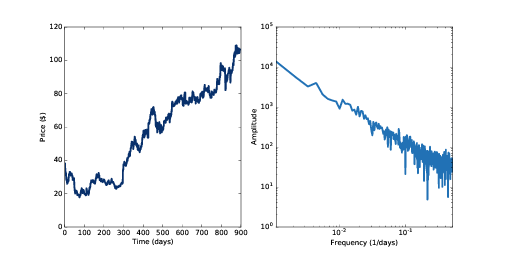diff = np.diff(ys)
change = thinkdsp.Wave(diff, framerate=1)
change_spectrum = change.make_spectrum()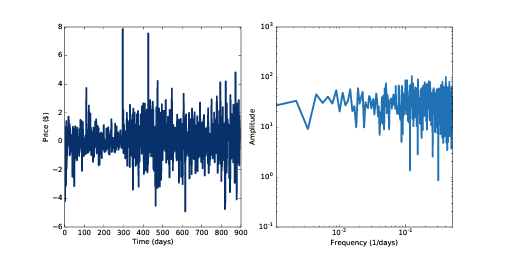## 9.2 频域¶

diff_window = np.array([1.0, -1.0])
diff_filter = diff_wave.make_spectrum()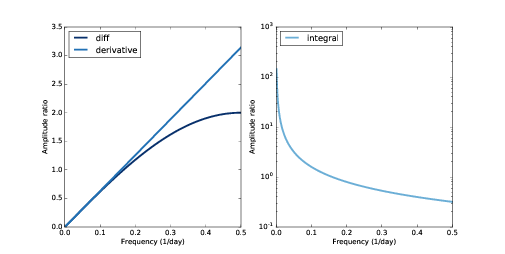## 9.3 微分¶

${E_f}(t) = {e^{2\pi ift}}$

$\frac{d}{{dt}}{E_f}(t) = 2\pi if{e^{2\pi ift}}$

$\frac{d}{{dt}}{E_f}(t) = 2\pi if{E_f}(t)$

deriv_filter = close.make_spectrum()
deriv_filter.hs = PI2 * 1j * deriv_filter.fs


7.4 所述，复指数信号乘以一个复数实际上产生了两种效果： 一是乘以一个幅值，这里是 $$2\pi f$$ ；二是移动一个初始相位，这里是 $$2\pi$$

• 算子是指从一个函数到另一个函数的映射关系。例如微分就是一个算子。
• 对于算子 A 和函数 g ，如果将 A 应用到 g 的结果 与 g 本身乘以一个标量值 $$\lambda$$ 相等，即 $$Ag = \lambda g$$ ， 那么我们称 gA 的特征函数。
• 相对应的，我们称标量 $$\lambda$$ 为特征函数 g 的特征值。
• 一个给定的算子可以有多个特征函数，每个特征函数均对应一个特征值。

1. 将信号表示成不同频率的复指数信号之和。
2. 计算每个频率成分的微分（乘法）
3. 将不同频率的微分结果累加

spectrum 类中提供了一个方法来计算差分滤波:

# class Spectrum:

def differentiate(self):
self.hs *= PI2 * 1j * self.fs


deriv_spectrum = close.make_spectrum()
deriv_spectrum.differentiate()
deriv = deriv_spectrum.make_wave()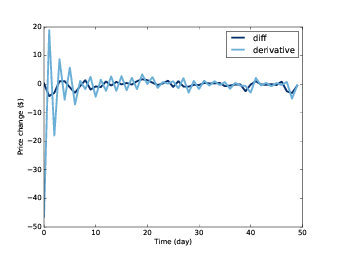• 计算相邻元素值之间的差分的方法，它又可以表示成对信号进行一个简单的卷积操作。最后的结果为一阶微分的近似值。
• 时域上的微分相当于频域上的一个滤波器。对于周期信号，它的结果是一阶微分，而对于非周期信号，它的结果近似于一阶微分。

## 9.4 积分¶

integ_filter = close.make_spectrum()
integ_filter.hs = 1 / (PI2 * 1j * integ_filter.fs)


Spectrum 类提供了一个方法来计算积分滤波:

# class Spectrum:

def integrate(self):
self.hs /= PI2 * 1j * self.fs


integ_spectrum = deriv_spectrum.copy()
integ_spectrum.integrate()


integ_spectrum.hs = 0
integ_wave = integ_spectrum.make_wave()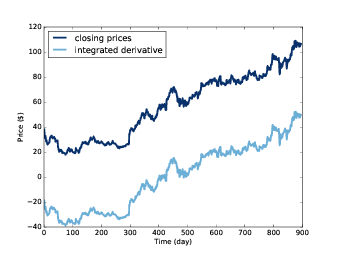## 9.5 累加¶

signal = thinkdsp.SawtoothSignal(freq=50)
in_wave = signal.make_wave(duration=0.1, framerate=44100)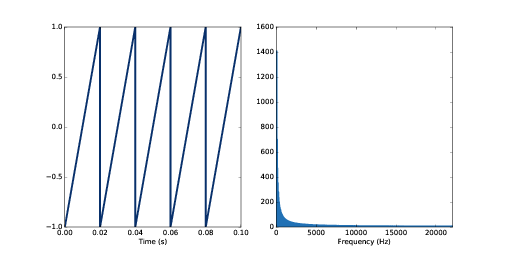Spectrum 类中提供了一个方法来计算波形的累加并返回一个新的波形:

# class Wave:

def cumsum(self):
ys = np.cumsum(self.ys)
ts = self.ts.copy()
return Wave(ys, ts, self.framerate)


out_wave = in_wave.cumsum()
out_wave.unbias()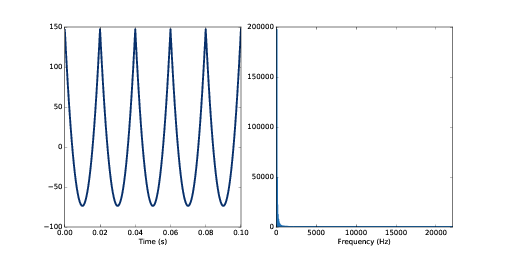cumsum_filter = diff_filter.copy()
cumsum_filter.hs = 1 / cumsum_filter.hs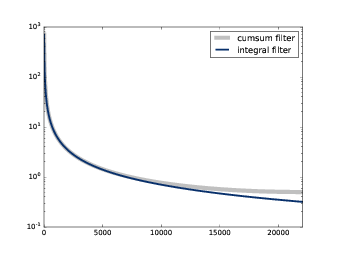in_spectrum = in_wave.make_spectrum()
out_spectrum = out_wave.make_spectrum()
ratio_spectrum = out_spectrum.ratio(in_spectrum, thresh=1)


def ratio(self, denom, thresh=1):
ratio_spectrum = self.copy()
ratio_spectrum.hs /= denom.hs
ratio_spectrum.hs[denom.amps < thresh] = np.nan
return ratio_spectrum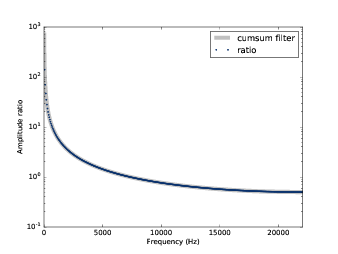out_wave2 = (in_spectrum * cumsum_filter).make_wave()


## 9.6 噪声的积分¶

4.3 中，我们通过对白噪声进行累加来计算出了布朗噪声，现在我们知道了累加操作 在频域上的效果，因此现在我们可以更深入的看一下布朗噪声的频谱。

${P_f} = K/{f^2}$

$\log {P_f} = \log K - 2\log f$

9.1 中，我们画出了Fackbook收盘价的频谱图，并得出它的斜率约为-1.9，这和布朗噪声是基本一致的， 其实很多股票的价格都有类似的频谱。